# Knowing the content

This section provides resources to improve teacher knowledge about the mathematics content covered in the Australian Curriculum: Mathematics.

Teachers can also improve their content knowledge by selecting a relevant free, self-paced online course provided by the University of Adelaide. Details for registration coming soon.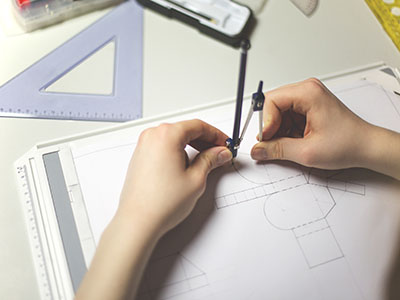## Primary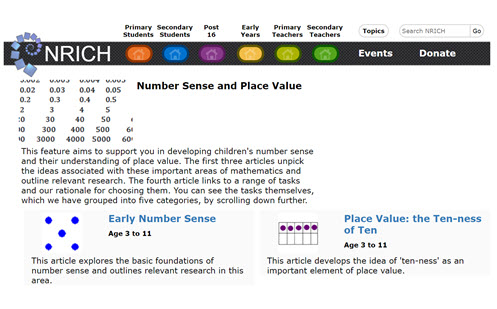Number sense and place value

A collection of brief articles that support teachers in developing students’ number sense and their understanding of place value.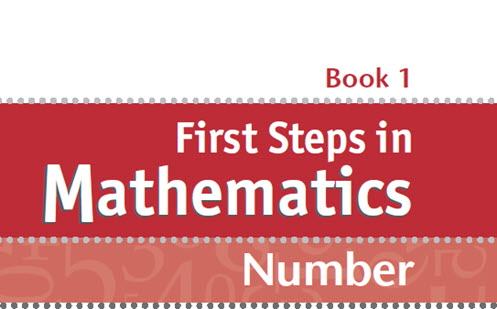First STEPS in mathematics: Number – Book 1

Content is organised into topics including: understanding whole and decimal numbers, and understanding fractional numbers.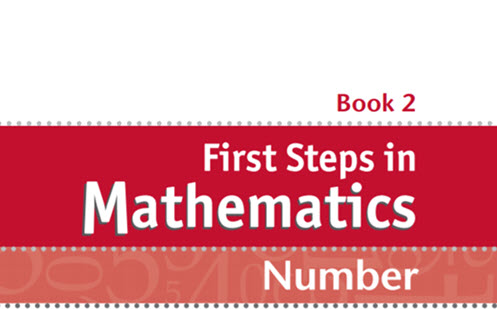First STEPS in mathematics: Number – Book 2

Content is organised into topics including: understanding operations, calculating, and reasoning about number patterns.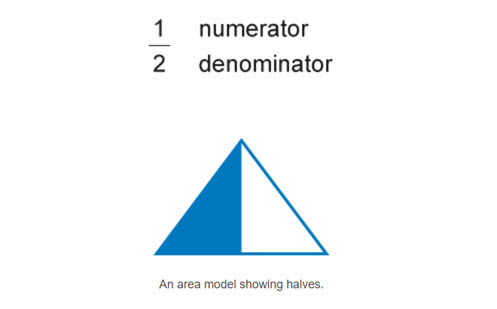Fractions: Big ideas

A fraction is often explained as a way of expressing 'parts of a whole'. While the part-whole idea describes a common use of fractions, it is not the only meaning. This resource explains the part-whole idea and also explains fractions as a number positioned on a number line, as well as the use of fractions to express division.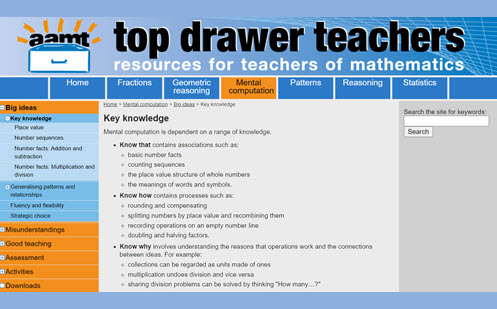Mental computation

Key knowledge is organised into topics: place value, number sequences, number facts, addition and subtraction, multiplication and division.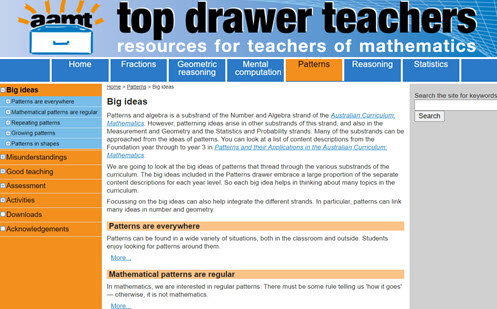Patterns: Big ideas

The concept of patterning is described as part of the number and algebra strand, and also the measurement and geometry strand, and the statistics and probability strand.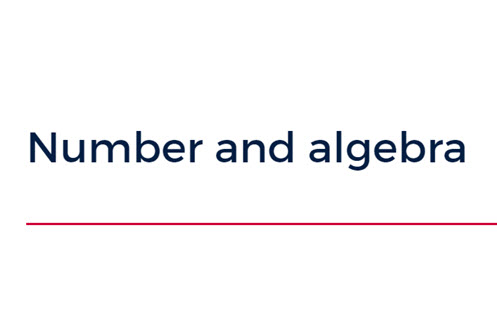NSW DET guide: Number and algebra

Content for number and algebra organised into topics and year levels (stages) incorporating examples of relevant teaching strategies.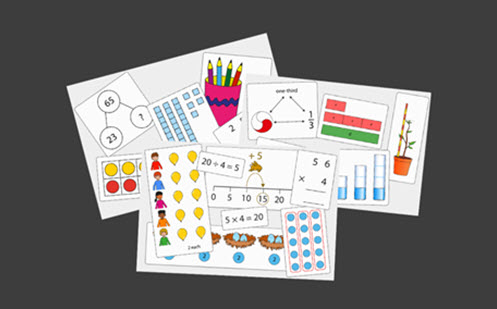Primary mastery professional development: Number

Subject knowledge is key to successful teaching. In this resource, the curriculum is split into a small number of areas, called ‘spines’:

• Spine 1: Number, Addition and Subtraction
• Spine 2: Multiplication and Division
• Spine 3: Fractions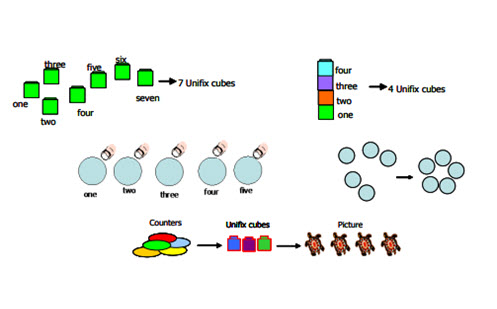Number: Foundation to Year 9

A comprehensive resource that describes the progression of number-related ideas showing the relationship to other strands.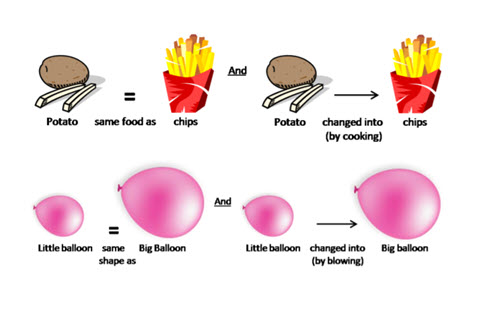Algebra: Foundation to Year 9

A comprehensive resource that describes the progression of algebra-related ideas and algebraic thinking.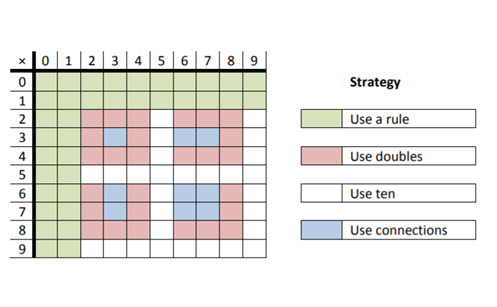Operations: Foundation to Year 9

A comprehensive resource that describes the progression of ideas that covers addition and subtraction of integers; multiplication and division of integers; the four operations with common and decimal fractions; and operation applications with percent, rate and ratio.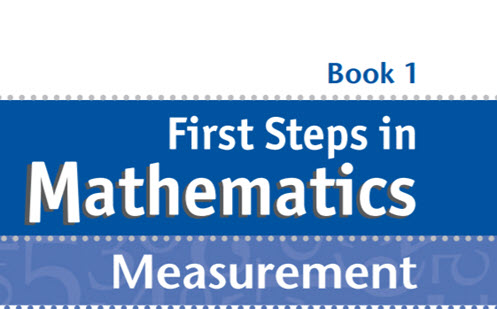First STEPS in mathematics: Measurement – Book 1

Content is organised into topics including: understanding units, and direct measuring.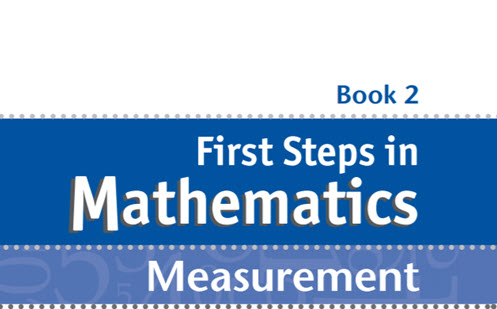First STEPS in mathematics: Measurement – Book 2

Content is organised into topics including: indirect measuring, and estimating.First STEPS in mathematics: Space

This guide supports teachers to develop students’ geometric understandings.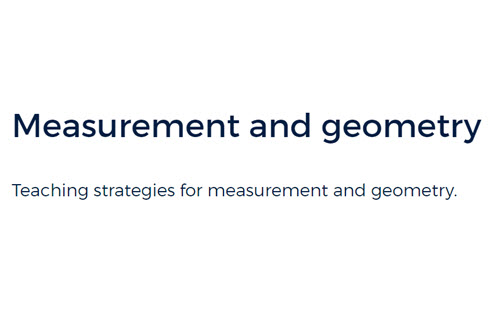NSW DET guide: Measurement and geometry

Content for measurement and geometry organised into topics and year levels (stages), incorporating examples of relevant teaching strategies.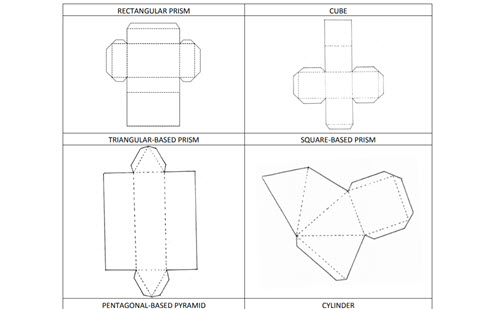Geometry: Foundation to Year 9

A comprehensive resource that describes the progression of geometric reasoning.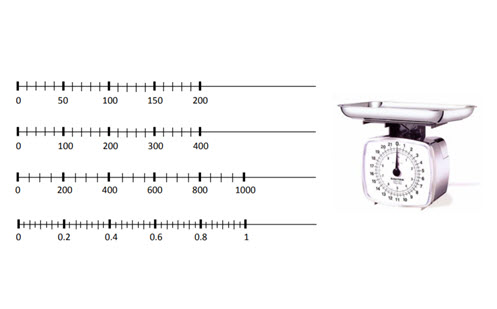Measurement: Foundation to Year 9

A comprehensive resource that describes the progression of measurement ideas showing the relationship to other strands.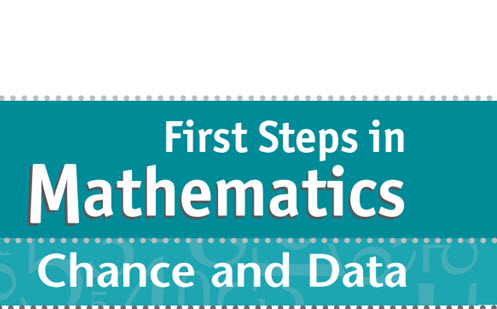First STEPS in mathematics: Chance and data

This guide supports teachers to develop students’ understanding, skills and processes related to probability and statistics.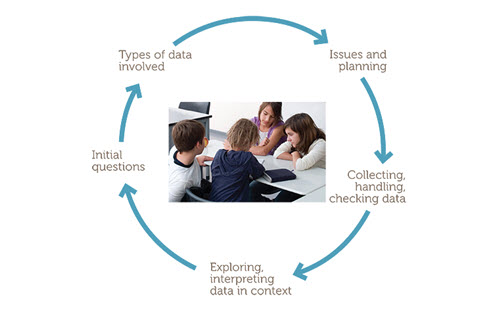Data investigation and interpretation (Year 5)

This resource provides a detailed overview of how to cover the content related to data investigation and interpretation for Year 5 students.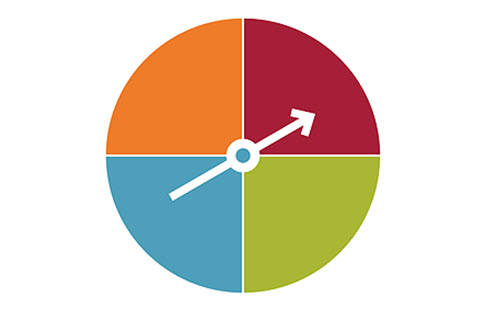Chance (Year 5)

This resource provides a detailed overview of how to cover the content related to chance for Year 5 students.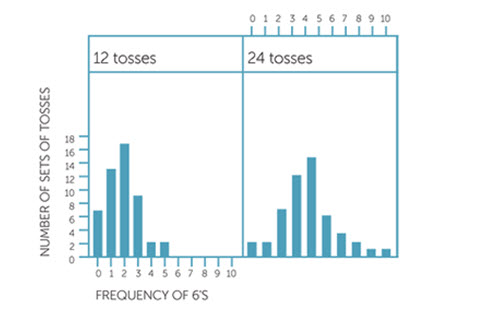Chance (Year 6)

A detailed overview of how to cover the content related to chance for Year 6 students.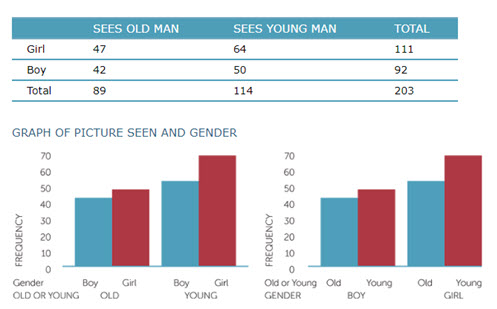Data investigation and interpretation (Year 6)

A detailed overview of how to cover the content related to data investigation and interpretation for Year 6 students.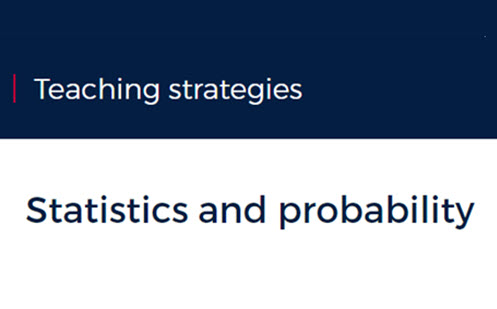NSW DET guide: Statistics and probability

Content for statistics and probability organised into topics and year levels (stages), incorporating examples of relevant teaching strategies.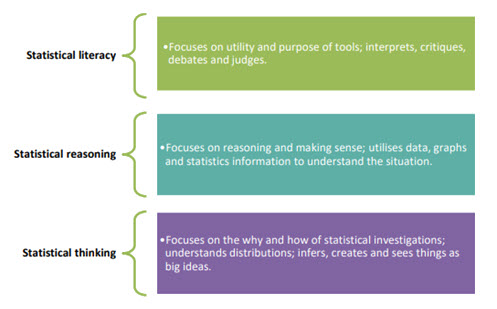Statistics and probability: Foundation to Year 9

Content for statistics and probability incorporating examples of relevant teaching strategies.

## Secondary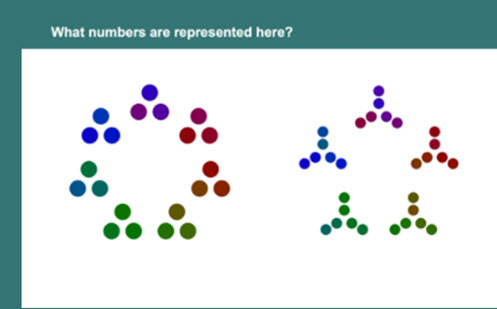Planning to teach secondary maths

This video and supporting resources provide advice about teaching number-related topics.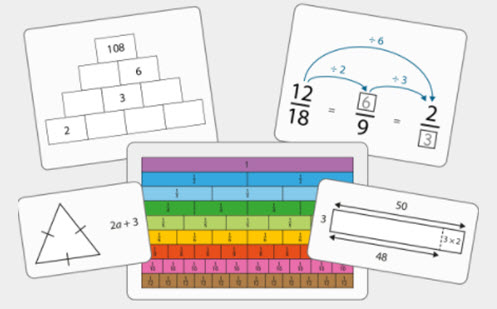Quantifying number (Years 7 and 8)

Details the structure of the number system for Year 7 and Year 8.Number: Foundation to Year 9

A comprehensive resource that describes the progression of number-related ideas showing the relationship to other curriculum strands.Operations: Foundation to Year 9

A comprehensive resource that describes the progression of ideas, covering areas including addition and subtraction of integers; multiplication and division of integers; the four operations with common and decimal fractions; and operation applications with percent, rate and ratio.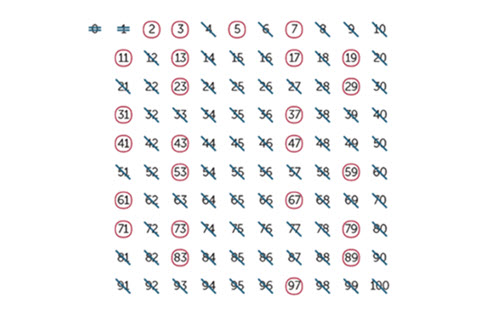Primes and prime factors (Year 7)

An explanation of primes, prime factors and finding the LCM and HCF.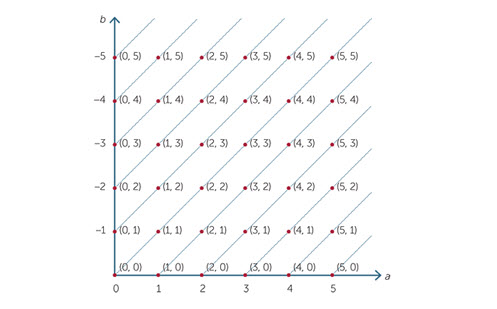Integers (Year 7)

Outlines how the order of operations effects positive and negative numbers.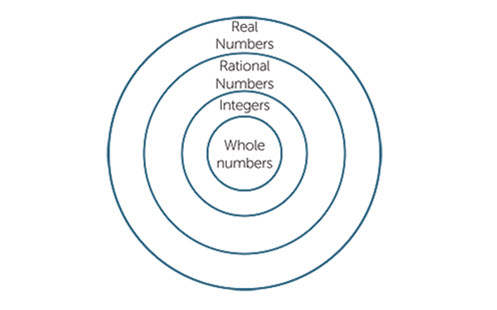Real numbers (Years 8, 9 and 10)

Details rational numbers, recurring decimals and surds.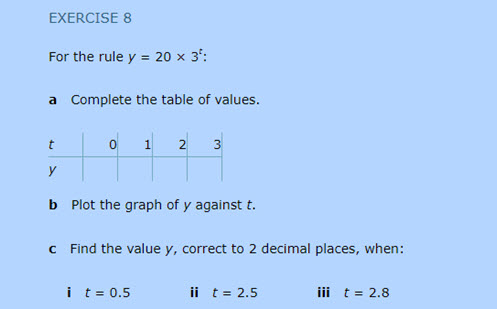Indices and logarithms (Years 9, 10 and 10a)

Expanding index laws to fractions and negatives, scientific and exponential notation.

### Fractions, decimals and percentages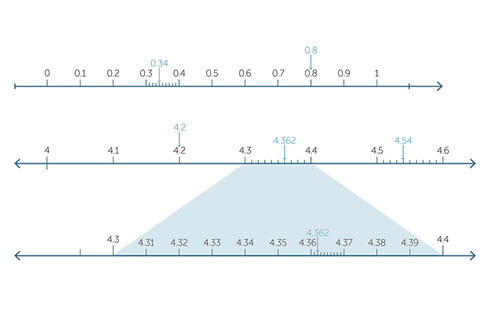Decimals and Percentages (Year 7)

Place value, operations with decimals and the connection to percentages.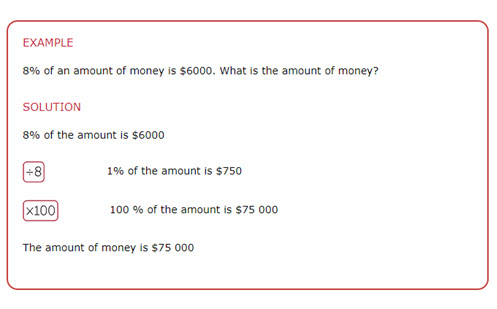Percentages (Years 7 and 8)

Connection of percentage to decimals, and fractions and operations using percentage.

### Rate and ratioThe unitary method (Years 7 and 8)

Explaining what the unitary method is and how to use it to solve problems.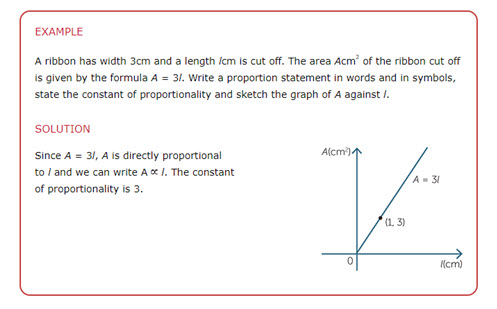Proportion (Years 9 and 10)

Explains what direct proportion is and how to illustrate it graphically.

### Financial maths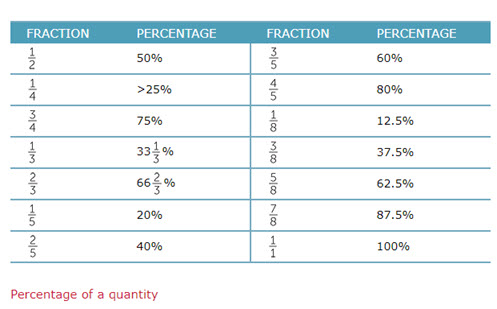Consumer arithmetic (Year 9)

Explains profit and loss to compound interest.

### Algebraic expressions and equationsAlgebra: Foundation to Year 9

A comprehensive resource that describes the progression of algebra-related ideas and algebraic thinking.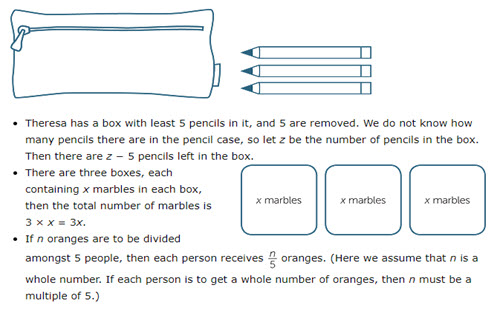Introduction to algebraic expressions (Years 7 and 8)

A comprehensive introduction on how to introduce pronumerals and write expressions from word problems, manipulate pronumerals and substitution.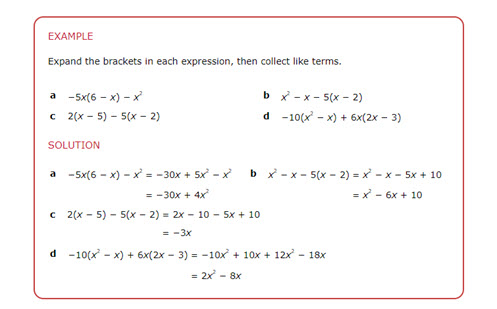Negatives and the index laws in algebra (Years 8, 9 and 10)

From simplifying expressions to using the index laws with pronumerals.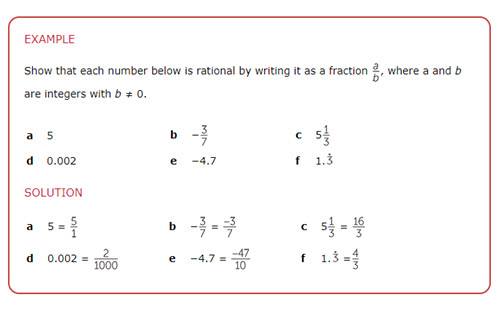Fractions and the index laws in algebra (Years 8 and 9)

Using pronumerals with fractions, decimals and powers.Special expansions (Years 9 and 10)

Expanding brackets and algebraic fractions.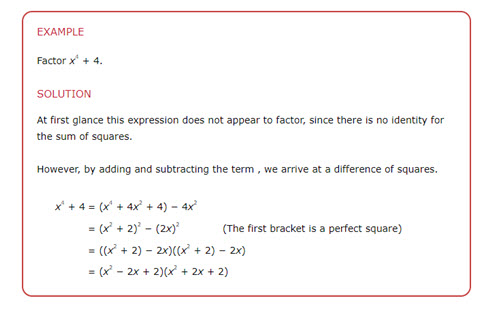Factorisation (Years 9 and 10)

Factorising quadratics and other polynomials.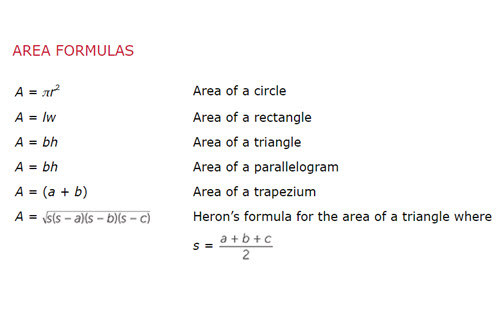Formulae (Years 9 and 10)

The meaning of a formula and substitution.

### Linear relationships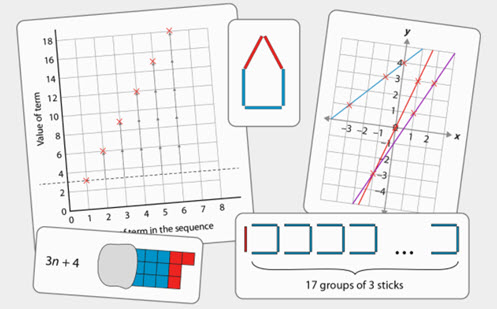Sequences and graphs (Years 7 and 8)

This unit explores the connections between patterns that form a sequence and their corresponding graphical representations.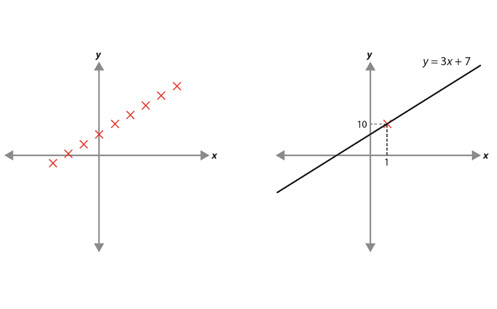Solving linear equations (Years 7 and 8)

How to solve linear equations, including equations with fractions and how to use identities.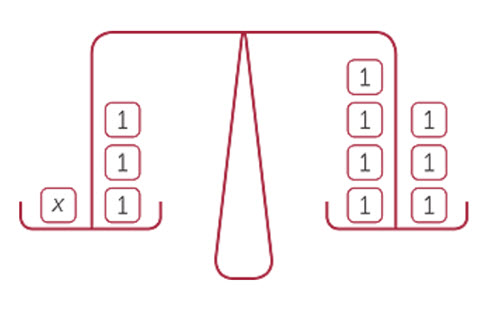Linear equations (Years 7, 8 and 9)

How to solve linear equations, including equations with fractions and how to use identities.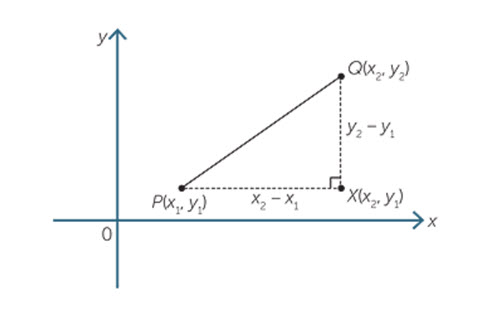Coordinate geometry (Years 9 and 10)

How to find the mid-point and length of a line and investigating different gradients.

### Non-linear relationships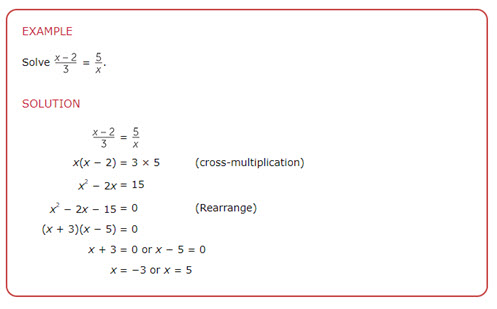Quadratic equations (Years 9 and 10)

Forming and solving quadratic equations.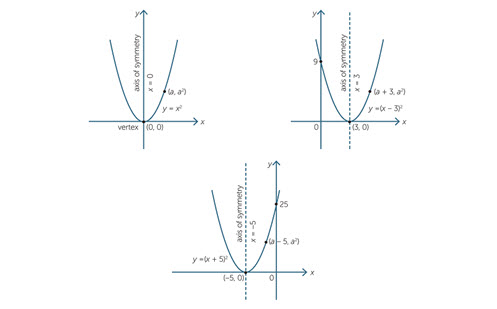The quadratic function (Year 10)

Graphing and transforming the quadratic function.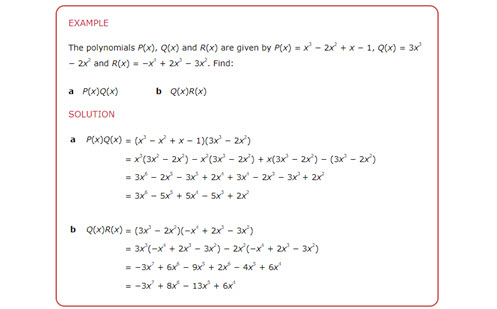Polynomials (Year 10)

Forming, factorising and graphing polynomials.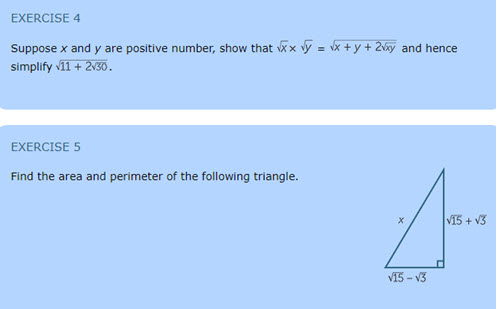Surds (Year 10a)

Simplifying and using surds.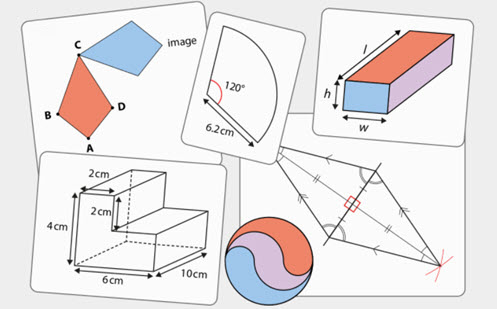Geometry and measurement: The basics

These units describe the basics of geometry and measurement.

### Units of measurementMeasurement: Foundation to Year 9

A comprehensive resource that describes the progression of measurement ideas showing the relationship to other strands.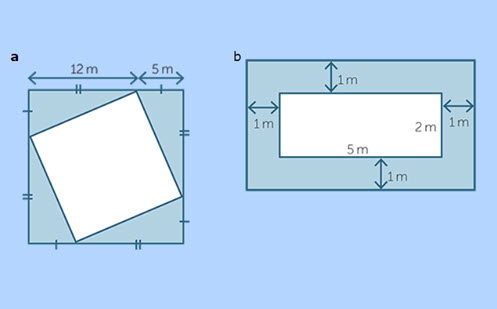Measurement (Years 7 and 8)

Units of measurement, perimeter and of rectangles and triangles.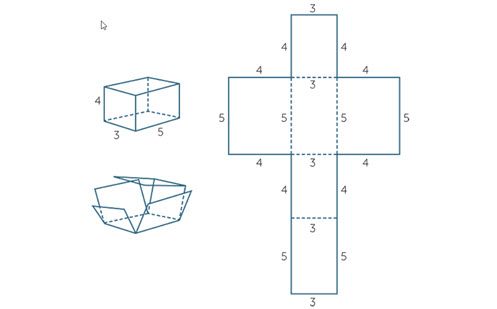Volume and surface area (Years 8 and 9)

Area of quadrilaterals, surface area and volume of prisms and cylinders.

### TimeTime (Years 8 and 9)

Exploring reading and measuring time, as well as timetables and time zones.

### Transformation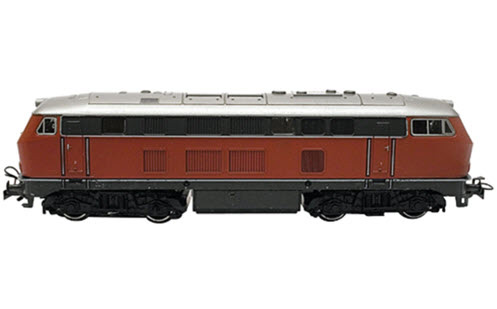Scale drawing and similarity (Years 8, 9 and 10)

Using and finding the scale of a diagram, and similarity tests for triangles.

### Geometric reasoningGeometry: Foundation to Year 9

A comprehensive resource that describes the progression of geometric reasoning.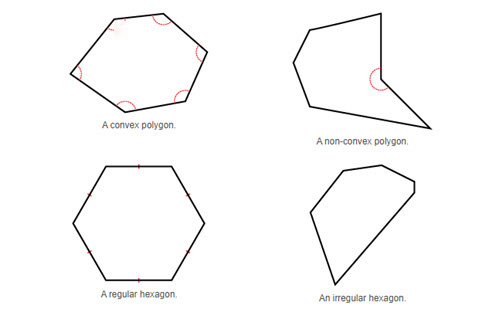Big Ideas in Geometric Reasoning (Years 7 to 10)

A look at the big ideas involved in developing geometric reasoning, and some of the misconceptions that students might hold.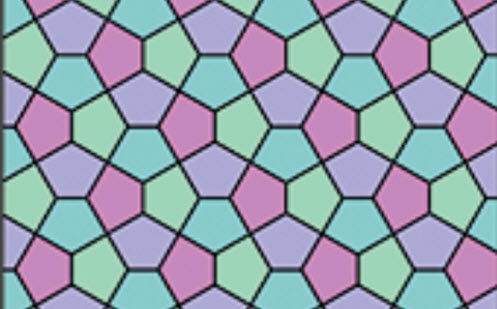Introduction to plane geometry (Years 7 and 8)

An introduction to the vocabulary of geometry and basic proofs.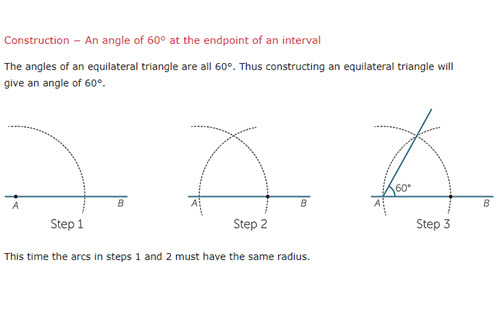Construction (Year 8)

How to use a pair of compasses and a ruler to construct angles and shapes.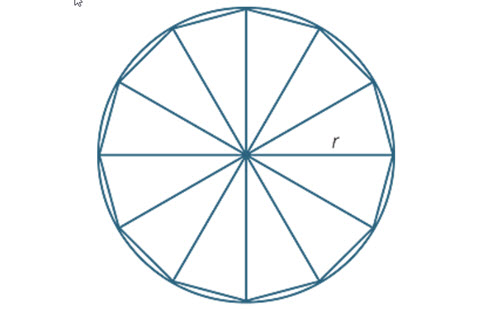Circles (Year 8)

How to introduce the vocabulary of circles and basic formulae.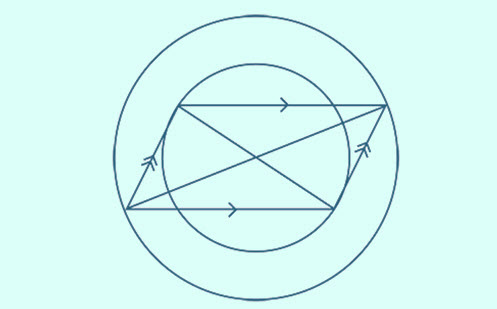Parallelograms and rectangles (Years 8 and 9)

The geometry of quadrilaterals.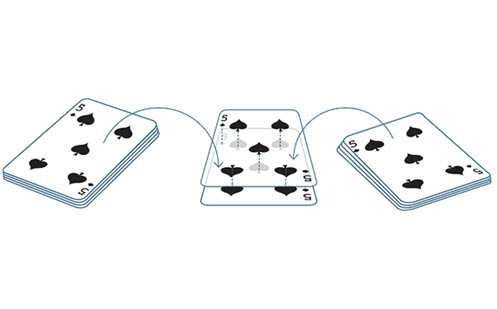Congruence (Year 8 and 9)

A resource describing what congruence is, and how to test for it.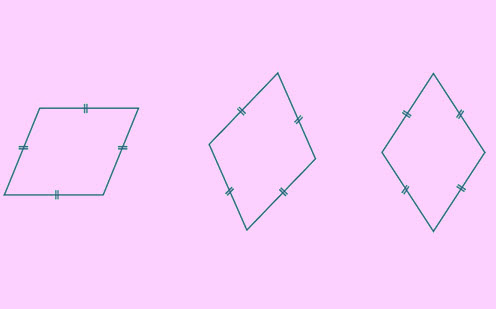Rhombuses, kites and trapezia (Years 8, 9 and 10)

Exploring the properties of these special quadrilaterals.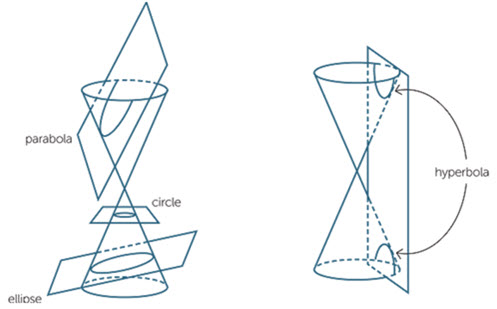Cones, pyramids and spheres (Years 9 and 10)

Looking at the surface area and properties of these 3D objects.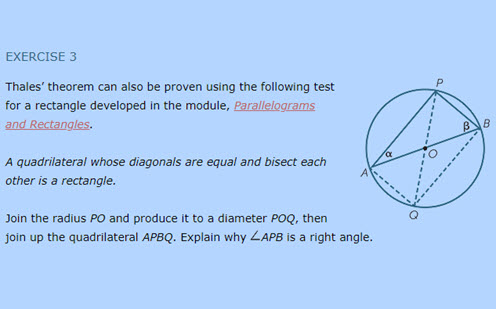Circle geometry (Year 9 and 10)

The geometric properties of circles, proofs and their uses.

### Pythagoras’ theorem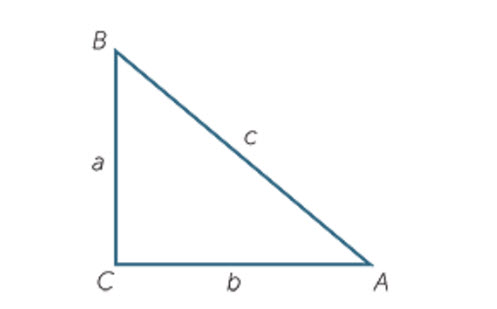Pythagoras’s theorem (Years 9 and 10)

Introducing the Pythagorean theorem and Pythagorean triads.

### Trigonometry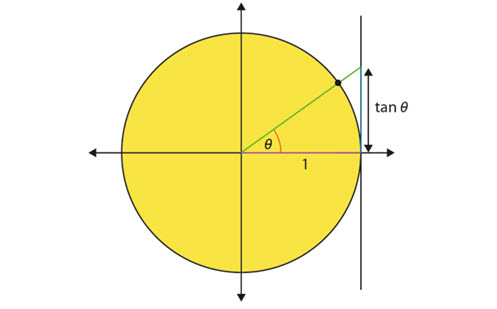Trigonometry (Years 9 and 10)

How to introduce trigonometrical vocabulary and identify misconceptions.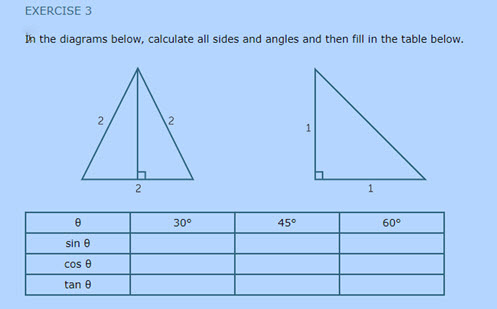Introductory trigonometry (Years 9 and 10)

Introducing trigonometrical ratios and their uses.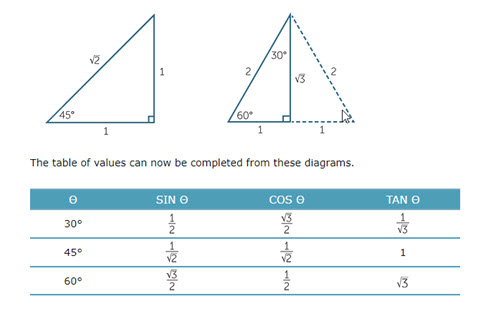Further trigonometry (Years 10 and10a)

Sine and cosine rules and their uses.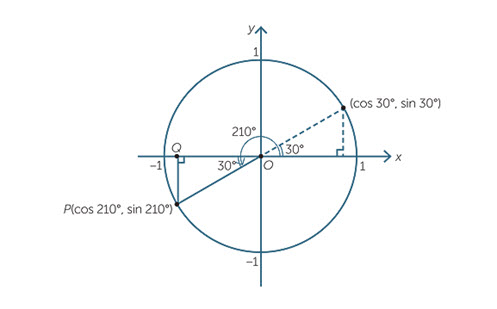Trigonometrical functions (Years 10 and 10a)

Looking at angles in all quadrants, introducing the unit circle.Statistics and probability: Foundation to Year 9

Content for statistics and probability incorporating examples of relevant teaching strategies.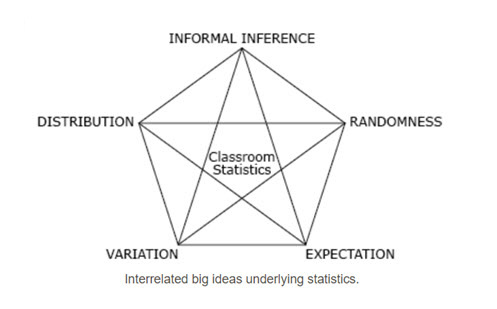Big ideas in statistics (Years 7 to 10)

A look at the big ideas involved in developing statistical understanding and some of the misconceptions that students might hold.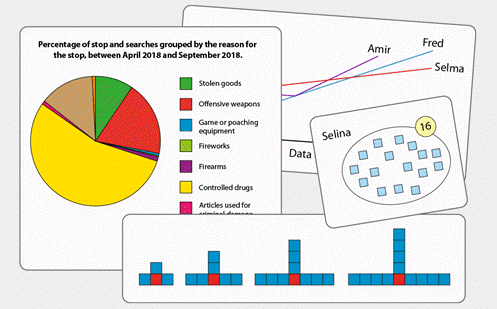Statistics and probability (Years 7 to 9)

These units explore the relationship between probability and the way data is represented.

### Probability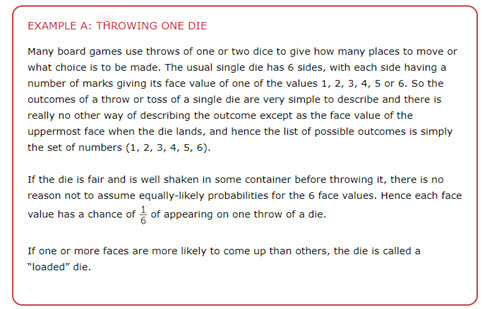Chance (Year 7)

Examining the meaning of equally-likely outcomes.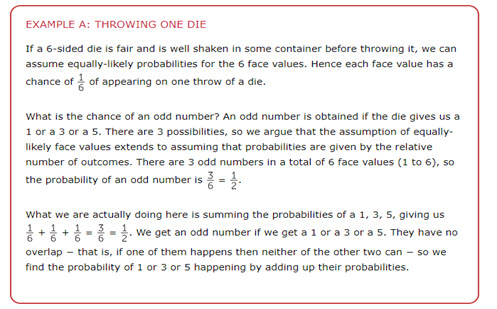Chance (Year 8)

Complementary events, and the meaning of the words ‘and’, ‘or’ and ‘not’.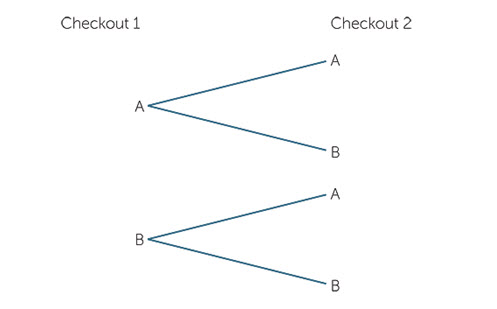Chance (Year 9)

Two-step experiments, estimating probabilities and using large sets of data.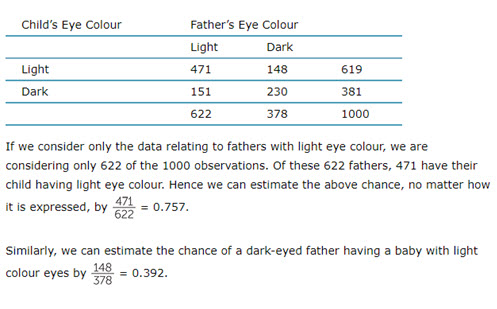Chance (Year 10)

Multistep experiments and the concept of independence.

### Data collection and representation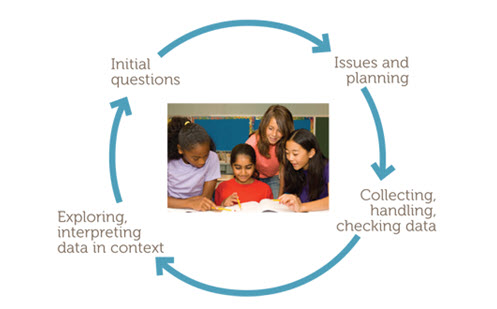Data representations (Year 7)

Collecting and interpreting data, and introducing stem and leaf plots.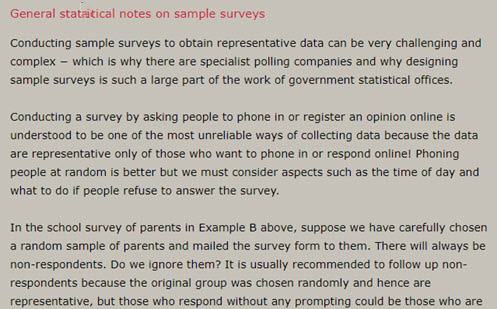Data representations (Year 8)

Types of data and variation across data sets.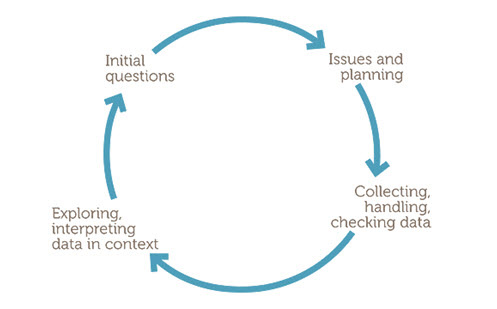Data representations (Year 9)

Introducing histograms and the concept of skewness.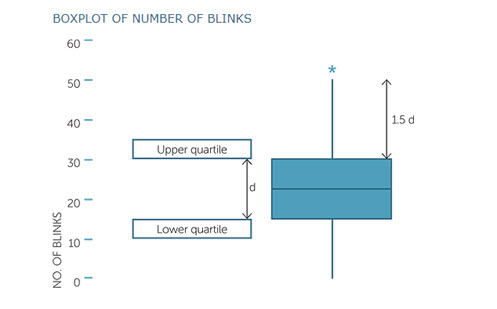Data representations (Year 10)

Introducing quartiles, boxplots and scatter plots.